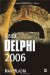Multidimensional Arrays

In Delphi, there are two ways of declaring multidimensional arrays:

ArrayName: array[Range_1, Range_2] of DataType;  ArrayName: array[Range_1] of array[Range_2] of DataType;

Just as one-dimensional arrays make it easier to work with large numbers of variables, multidimensional arrays help us when we have to work with a large number of one-dimensional arrays. For instance, we can use a very simple two-dimensional array to hold the multiplication table and then read appropriate values when we have to.

Listing 6-6: A simple two-dimensional arrayprogram Project1; {\$APPTYPE CONSOLE} uses   SysUtils; var   MultTable: array[1..10, 1..10] of Integer;   i, j: Integer; begin   for i := 1 to 10 do   begin     for j := 1 to 10 do     begin       MultTable[i, j] := i * j;       Write(MultTable[i][j]:4);     end;     WriteLn;   end;   ReadLn; end.The example in Listing 6-6 illustrates both ways of accessing a value in a multidimensional array:

ArrayName[index_1, index_2]  ArrayName[index_1][index_2]Inside Delphi 2006 (Wordware Delphi Developers Library)
ISBN: 1598220039
EAN: 2147483647
Year: 2004
Pages: 212